• Save

# Mixture and Alligation Problems with Solutions for Competitive Exams

9 months ago 2.0K ViewsMixture and Alligation is an important topic of a quantitative aptitude. In this topic, I am giving you rules to solve questions of mixture and alligation which will help you to prepare for competitive exams like SSC and Bank exams.

Here are the problems and solutions of mixture and alligation for your practice in this topic which helps you to understand topic properly. You can visit on Alligations and Mixtures Exercise to Practice more for a better rank in the competitive exams.

## Solutions of Mixture and Alligation Problems

### What is the Mixture?

Mixture

The new product by mixing two or more ingredients in a certain ratio in called a mixture of those particular ingredients.

Mean Price

The cost price of a unit quantity of the mixture is called the mean price

Rules of Mixture or Allegation

→ This rule of mixture is use to determine the mean price of the mixture, when the price of the individual items being mixed together and the proportion in which they are being mixed are given.

→ When two ingredients at given prices are known, then the ratio in which two are mixed to obtain a mixture of known price is given by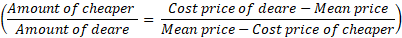It is also known as rule of allegation. It can also be expressed, as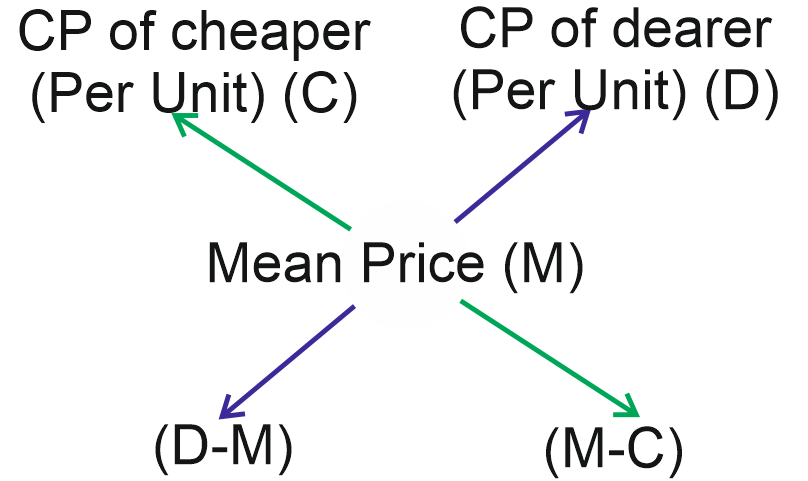Hence, Amount of cheaper: Amount of dearer = (D-M): (M-C)

Note.1. The value of the mixture is always higher than the lowest value and lower than the highest value of the items being mixed.

Note.2. Rule of mixture or allegation is applied for rate, ratio, percentage value, speed, price etc. and not for absolute values. In other words, whenever percent. Per hour, per km, per kg etc. are being compared, we can use this method.

Q.1. In what ratio must a grocer mix two varieties of tea costing Rs. 15 and Rs. 20 per kg respectively, so as to get a mixture worth Rs. 16.50 per kg?

(A) 3 : 7

(B) 5 : 7

(C) 7 : 3

(D) 7 : 5

Ans .   C

Accordint to the allegation rule,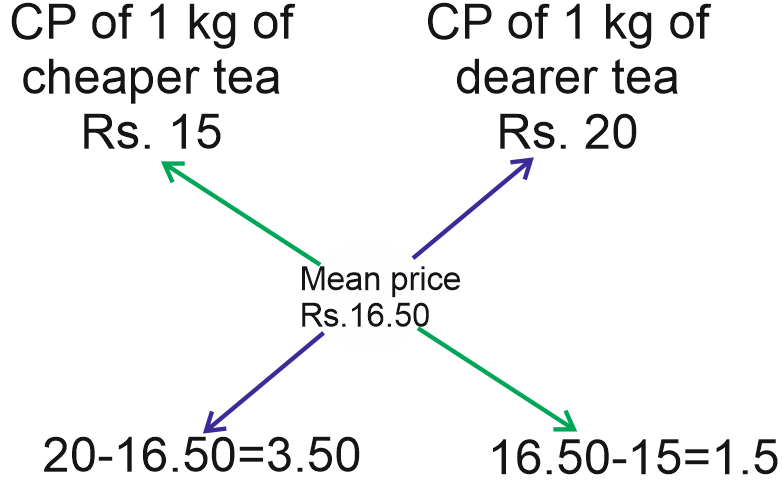Now, required ratio =

(cheaper tea) : (Dearer tea)

= 3.50 : 1.5 = 350 : 150 = 7 : 3

Q.2. A mixture of certain quantity of milk with 8 L of water is worth 45 paisa per litre. If pure milk be worth 54 paisa per litre, how much milk is three in the mixture?

(A) 40 L

(B) 35 L

(C) 25 L

(D) 45 L

Ans .   A

According to the rule of allegation,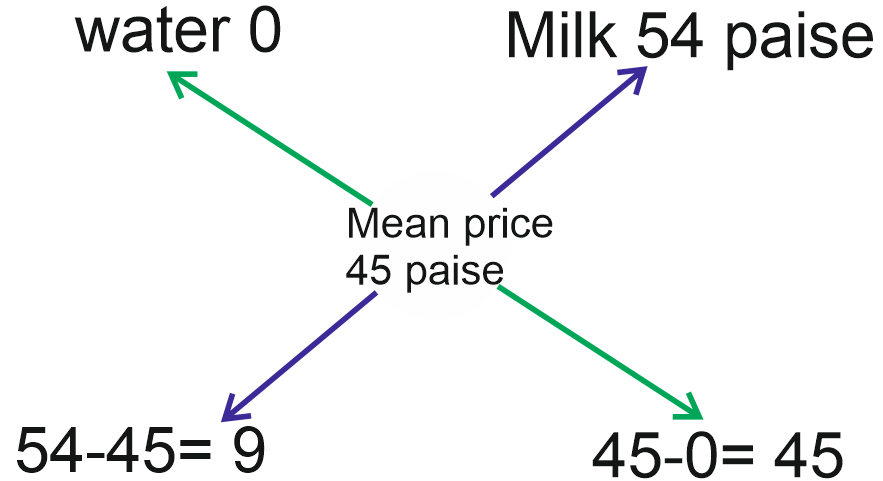⸫ Water : Milk = 9 : 45 = 1 : 5

Hence, quantity of milk in the mixture

= 5×8 = 40 L

Q.3. A trader has 50 kg of pulse, part of which he sells at 8% profit and rest at 18% profit. He gains 14% on the whole. What is the quantity sold at 18% profit?

(A) 30 kg

(B) 35 kg

(C) 40 kg

(D) 60 kg

Ans .   A

According to the rule of allegation,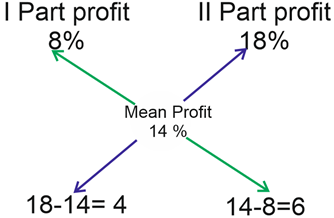⸫ (I Part) : (II Part) = 4 : 6

= 2 : 3

Hence, quantity sold at 18% profit

= (3/5)×50 = 30 kg

Q.4. 600 g of sugar solution has 40% sugar in it. How much sugar should be added to make it 50% in the solution ?

(A) 160 g

(B) 120 g

(C) 130 g

(D) 140

Ans .   B

The existing solution contains 40% sugar and here sugar has to be mixed. Therefore, the other solution has 100% sugar.

According to the rule of allegation,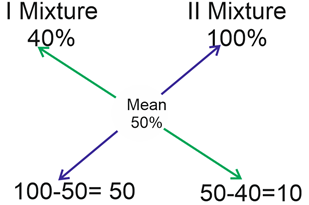⸫ Required ratio = 50 : 1.

= 5 : 1

So, the two mixtures should be added in the ratio of 5 : 10.

Hence, required sugar = (600/5)×1 = 120 g

Q.5. Two vessels P and Q contain wine and water in the ratios of 5:2 and 8 : 5 respectively. Find the ratio in which these mixtures are to be mixed to get a new mixture containing wine and water in the ratio of 9: 4.

(A) 2 : 3

(B) 7 : 2

(C) 7 : 3

(D) 3 : 7

Ans .   B

Let the CP of wine = Rs 1 per litre

Wine in 1 L Mixture of P = 5/7 L

Wine in 1 L Mixture of Q = 8/13 L

Wine in 1 L Mixture of P and Q = 9/13 L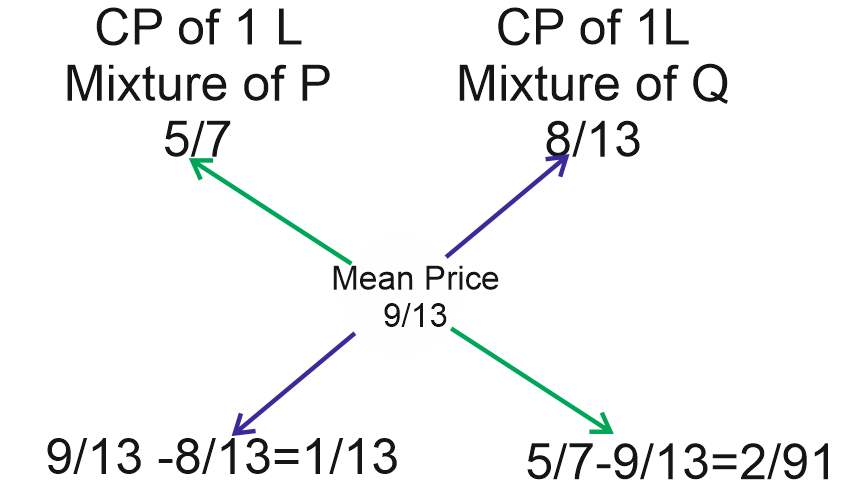CP of 1 L Mixture of P = Rs 5/7

CP of 1 L Mixture of Q = 8/13

Mean Price = Rs 9/13

⸫ Required ratio = 1/13 : 2/91 = 1: 2/7 = 7 : 2.

Q.6. In what ratio must a grocer mix rice worth Rs. 30 per kg, so that by selling the mixture at Rs. 34.10 per kg, he may gain 10%?

(A) 3 : 4

(B) 3 : 2

(C) 3 : 5

(D) 5 : 4

Ans .   B

Given, selling price (SP) of 1 kg

Mixture = Rs.34.10

Gain = 10%

⸫ Cost price (CP) of 1 kg mixture = (100/110)×34.10 = Rs.31.

Now, according to the rule of allegation,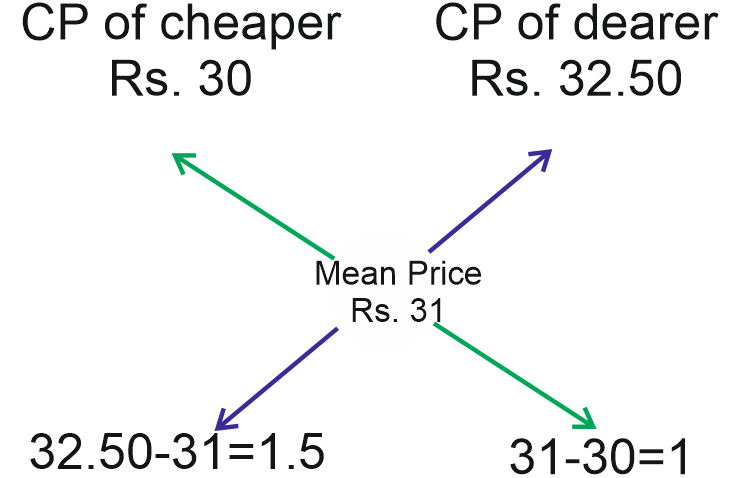⸫ Required ratio = 1.5:1 = 3 : 2.

Q.7. There are 65 students in a class, Rs. 78 are distributed among them so that each boy gets Rs. 1.6 and each girl gets Rs. 0.60. Find the numbers of boys and girls in the class.

(A) 26, 39

(B) 27, 38

(C) 28, 37

(D) 39, 26

Ans .   D

Mean amount = 780/65 = 120 paise

According to the rule of allegation,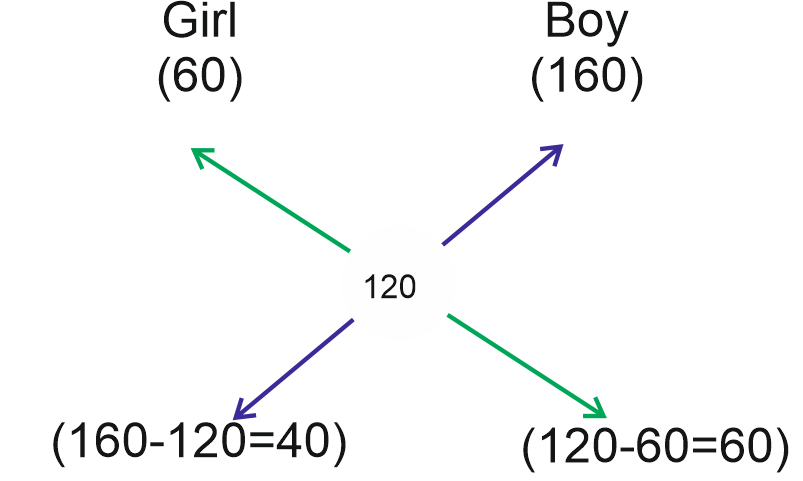Girl : Boy = 40 : 60 = 2 : 3

⸫ Number of girls = (2/5)×65 = 26

And number of boys = (3/5)×65=39

Q.8. A butler stole wine from a butt of sherry which contained 80% of spirit and he replaced it by wine containing only 32% spirit. Then, the butt was of 48% strength only. How much of the but did the steal?

(A) 1/4

(B) 3/5

(C) 2/5

(D) 2/3

Ans .   D

According to the rule of allegation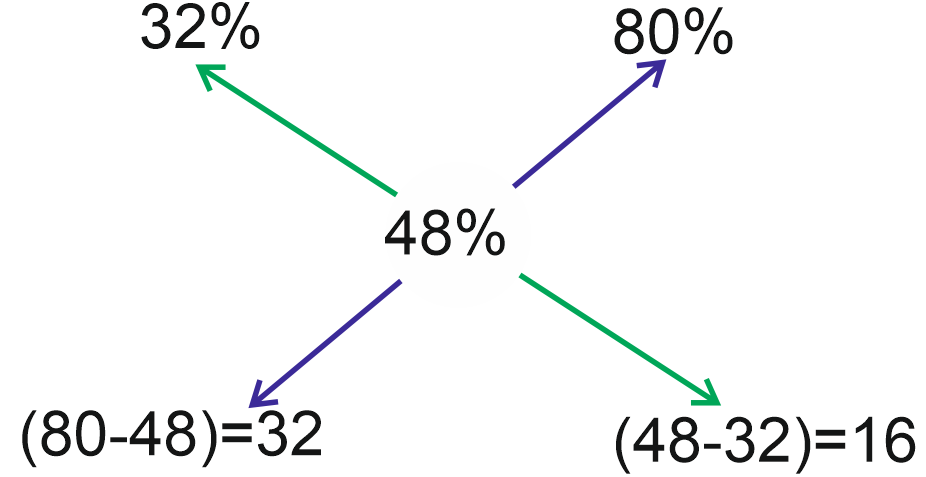⸫ Required ratio = 32 : 16 = 2 : 1

Clearly, 1/3 of the butt of sherry was left and the butler stole 2/3 of the butt.

Q.9. A mixture contains milk and water in ratio 4 : 3. If 5 L of water is added to the mixture, the ratio becomes 4 : 5. The quantity of milk

(A) 10 L

(B) 11 L

(C) 15 L

(D) 16 L

Ans .   A

Required quantity = (4×5)/(5-3)= 20/2= 10L

Q.10. A container has 60 L of milk, from his container, 4 L of milk is taken out and replaced with water. If this process is repeated 3 times, then quantity of milk in container left.

(A) 568.7 L

(B) 754.6 L

(C) 48.78 L

(D) None of these.

Ans .   C

Required quantity = 60{1-(4/60)}3= 60{(15-1)/15}3

= 60×{(14)3/(15)3} = 48.78 L

Q.11. On combining two groups of students having 30 and 40 marks respectively in an exam, the resultant group has score of 34. Find the ratio of the number of students in the first group to the number of students in the second group

(A) 2 : 1

(B) 3 : 2

(C) 3 : 1

(D) 4 : 3

Ans .   B

According to the allegation rule,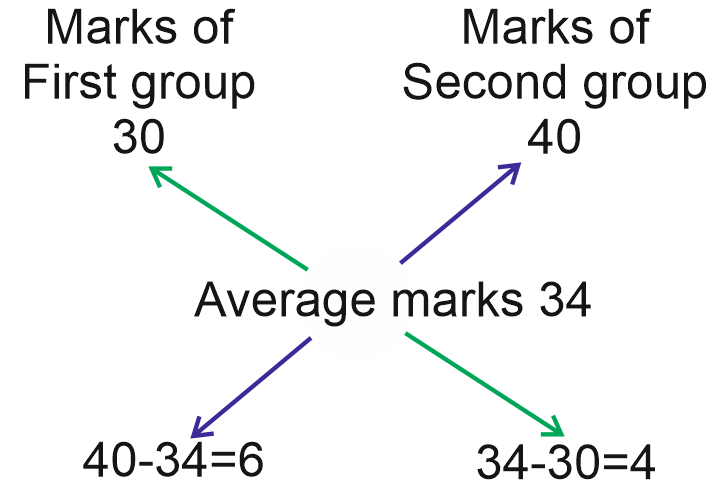⸫ Required ratio = 6 : 4 = 3 : 2